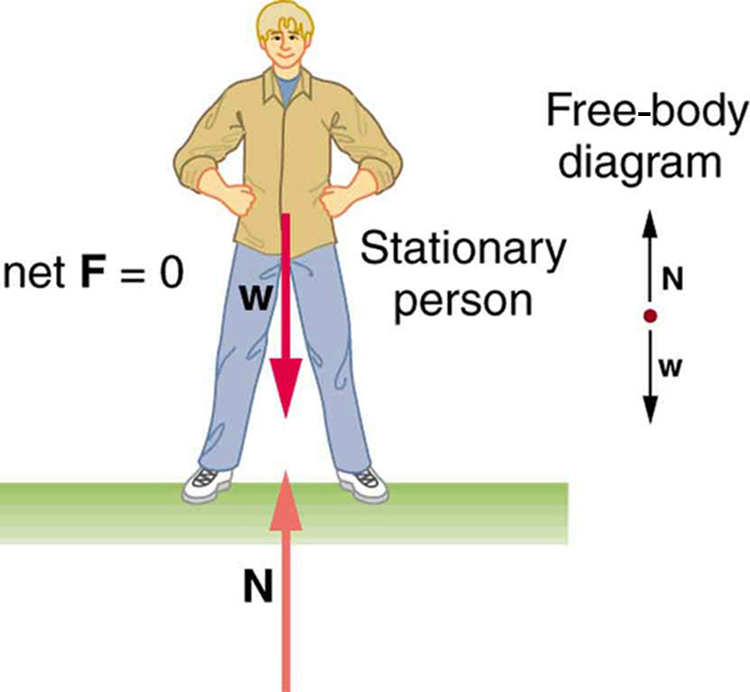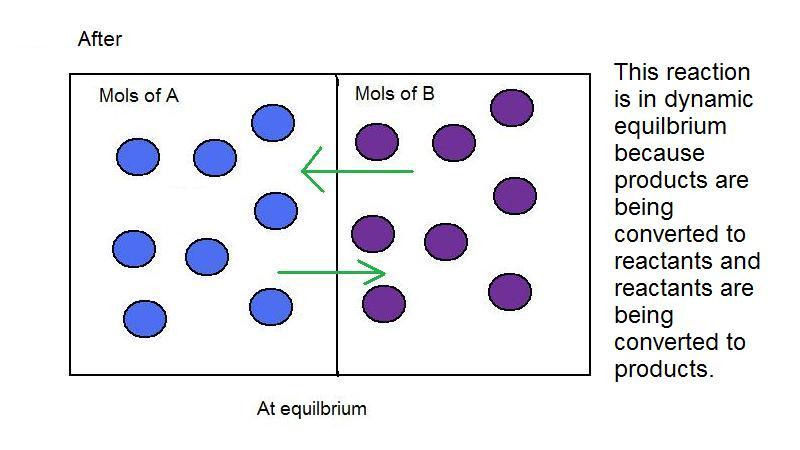Difference between Static and Dynamic Equilibrium

There are plenty of differences between two types of equilibrium – static and dynamic.

The biggest difference is that in static equilibrium, the object is at rest while in dynamic equilibrium it is moving with a constant velocity, be it angular or linear.

In static equilibrium, there is no change in the microscopic and macroscopic properties of the material on which the forces or moments are acting, whereas in dynamic equilibrium, the microscopic properties of the material change.

Dynamic equilibrium has applications in thermodynamics and chemistry as well. The same cannot be said about the static equilibrium. In the thermodynamic case, if the rate of inflow of mass, heat or energy is equal to the outflow, the system is in dynamic equilibrium. In chemistry, if the rate of forward reaction is equal to the rate of back ward reaction, the system is in dynamic equilibrium.

Instructions

• 1

Static Equilibrium

A system is in equilibrium when the sum of all the forces and the moments acting on it is zero.

In static equilibrium, the velocity of the system or the rate of change in displacement is also zero. Thus the object is static and there is no change in the microscopic and macroscopic properties whatsoever.

For example, consider a case of glass, full of water, which is lying on the table. The force acting on the table depends on the amount of water in the glass and the gravitational pull of the earth. To neutralize this force and keep the glass in equilibrium, the table exerts a reaction force on the glass. Thus the glass remains in equilibrium with the table. As the glass in motionless, we can deduce that it static equilibrium.

Image courtesy: cnx.org• 2

Dynamic Equilibrium

Dynamic equilibrium, is the type of equilibrium in which the sum of the input factors (force, moments, energy etc) is equal to the output, but the system is in motion. The rate of change of displacement is not zero, but the rate of change of velocity (acceleration) is zero.

Considering the same example of a glass lying in static equilibrium on the table; the system will be in dynamic equilibrium if the glass are table are lying in a train moving at a constant velocity.

In a thermodynamic system, a system may be moving with constant velocity due to work, but if the sum of energies at the input is equal to the sum of energies at the output, the system is in dynamic equilibrium.

Image courtesy: chemwiki.ucdavis.edu•